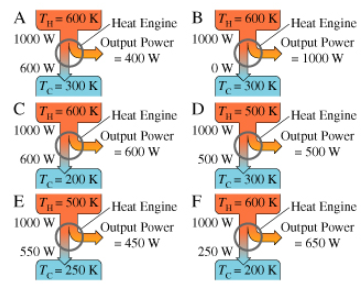# Problem: Which of the designs violate(s) the first law of thermodynamics?

###### FREE Expert Solution

The first law of Thermodynamics:

$\overline{){\mathbf{∆}}{\mathbf{U}}{\mathbf{=}}{\mathbf{Q}}{\mathbf{+}}{\mathbf{W}}}$

ΔU is the change in internal energy, Q is the heat transfer, and W is the amount of work done.

88% (310 ratings)###### Problem Details

Which of the designs violate(s) the first law of thermodynamics?